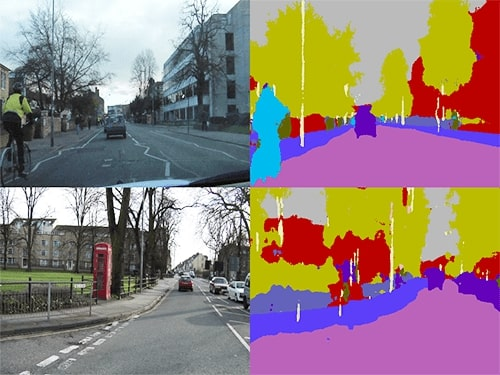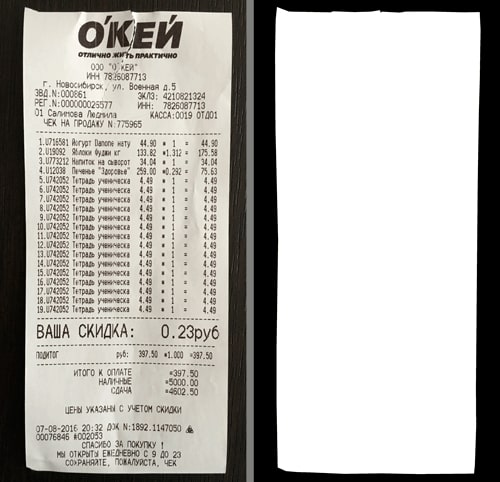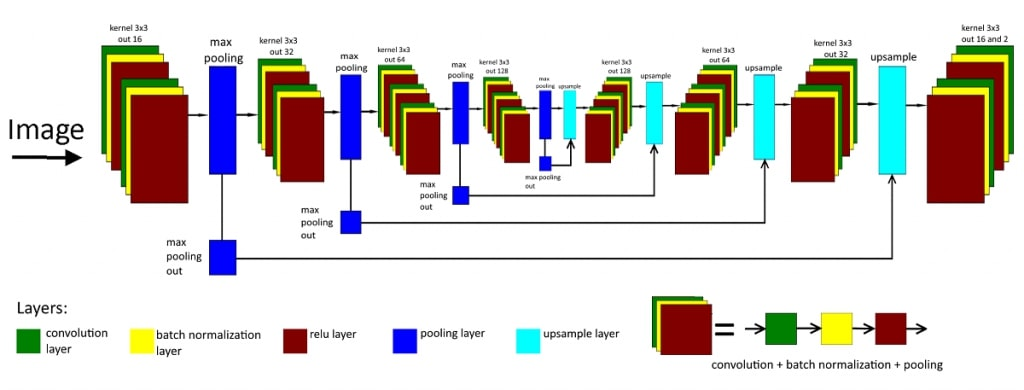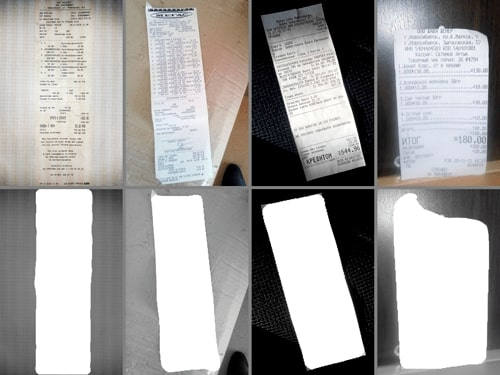# Fully Convolutional Networks for Semantic SegmentationIvan Ozhiganov

Founder & CEO at Azoft

30 Jun 202130 Jun 2021

Applying machine learning algorithms for object recognition solves many problems even more effectively than human eyes. For example, convolutional neural networks are most commonly used in tasks for image analysis including object detection, classification, and recognition. The range of these tasks is gradually becoming wider. Due to this, the development of new network architectures, layers, and framework modifications remains of current interest.

In our research, we often turn to methods using convolutional neural networks. In particular, we recently used a special type of neural network, fully convolutional neural networks for the receipt recognition project.

Now we’re going to tell you about the specifics of working with a fully convolutional network and the results we achieved.

## Research Overview

This research is implemented within a project devoted to receipt recognition. To achieve the goal we need to train a FCN to recognize where the object is — the receipt — and where the background is.

Our research includes the following stages:

• Studying the Specifics of Fully Convolutional Networks
• Comparing Fully Convolutional Networks and Convolutional Neural Networks
• Learning the Architecture of Fully Convolutional Networks
• Preprocessing
• Training the network for object detection

## Implementation

### 1. The Specifics of Fully Convolutional Networks

A FCN is a special type of artificial neural network that provides a segmented image of the original image where the required elements are highlighted as needed.For example, fully convolutional networks are used for tasks that ask to define the shape and location of a required object. These tasks seem to be complicated to accomplish using ordinary convolutional networks. To understand why and when FCN is better than CNN, we need to compare these types.

### 2. Comparing Fully Convolutional Networks and Convolutional Neural Networks

The most obvious difference between FCN and CNN is the outcome of their work. Ordinary convolutional neural networks can be used for classification to determine the class of some images and for object localization via regression.

As a result, the output data in any of these methods are numbers or arrays of numbers. In other words, we can get very restricted information about the image, but cannot transform it in the required view.

At the output, FCN gives a segmented image according to the size of the image at the input. Therefore, an alternative name of these networks is segmentation neural networks.

Segmentation is the process of combining objects in groups in accordance with the join features. Hence, we get a lot of information from the network and can process the outcome using simple heuristic methods.

### 3. Architecture of Fully Convolutional Networks

In order to understand the principles of how fully convolutional networks work and find out what tasks are suitable for them, we need to study their common architecture. While convolutional networks are being planned, we can add various layers to their architecture to increase the accuracy of recognition (drop out layer, local response normalization layer, and others). For now, we’re going to consider only the basic architecture that is almost unchangeable and defines how FCN works.

The convolution of the image makes up the backbone of how it works. The key layers are convolution layers. The convolution layer includes the number of outputs, the convolution kernel, the step of the layer, the size, and the indent.

A convolution operation passes with the kernel through the whole image. After that, we get the value of response to one or the other kernel in each point of the image. The number of kernels for every convolution layer equals the product of layers outputs to the number of input images. Then the received results go through the next convolution layer by getting the values for the other kernels. The regularization layer or normalization layer can be added to each convolution layer depending on what the developer chooses. When images go through many convolution layers, it helps researchers to get a wide variety of possible interpretations of the image.

When an image went through the required number of the convolution layer, it moves to the pooling layer. This layer reduces the size of input images without reducing their amount. The layer has a kernel that moves similarly to the convolution layer and calculates the only value for each image area. Image reduction speeds up the data processing in the network. It also allows adding more outputs in the following convolution layers and thus increasing the precision of the results. The point is that when the image is reduced, convolution layers of the same size can capture a greater part of the required object.

The sequence convolution/../../convolution/pooling (where the number of convolution layers is defined by the developer) can be repeated several times until we get a minimum image size. This size is determined experimentally.Highlighted objects will correspond to the original scale of the image if the reduced image turns back to the initial size. An upsample layer implements the image enlargement. Every output has two input images. The first is a processed image from the previous layer — convolution or pooling. The second is an image from the pooling layer, where the number of outputs equals the number of inputs of the correspondent upsample layer and the size of the output pooling image equals the size of the input upsample image.

Hence, we get symmetric architecture relative to the last pooling layer and the first upsample layer. There are convolution layers among layers of image enlargement, but the number of outputs from these layers reduces slowly.

The sequence upsample/convolution/../../convolution is needed to bring the image back to its initial size and to cut down the number of possible image interpretations to the number of the required object groups.

### 4. Preprocessing: Creating a Dataset for Building the Model of the Neural Network Architecture

We use the framework Caffe Segnet for implementing the algorithm of the fully convolutional network in our research. Caffe Segnet is a Caffe version that is developed specifically for FCN.

As per the research goal, we need to train our network for receipt recognition. To implement this we need to provide quite a large sample of images. Then a sample should be divided into parts in such a way that every image has its own mask.

A mask is a monochrome image, where every group of objects is determined by a specific color from 0 to 255. For example, in our case, there are two groups: the receipt and the background. The background has color 0, and the area of the receipt has color 1. The image below shows an example of the pair “image & mask”, and in order to see the marked receipt, its value is determined as 255.Thus, when we created a large dataset of similar images, we turned to the implementation of our network architecture model. The architecture is based on the principle of work of the segmentation neural networks.

### 5. Training the Network for Object Detection

We prepared a large dataset of images for training the network. It‘s easier if you split this dataset into two directories: the original and the mask. Let’s give every mask the same name as its original image counterpart. So that if you need to find an original/mask pair, the search algorithm will be easier. Then you need to add the text file including ways to pair images, where every pair can be determined as a “way/to/the image/original” and a “way/to/the image/mask”. One line implies one pair. Then you should give this file to the network to find the location of the input training data.First of all, we upload to the network the image and its mask. Then the training iteration begins – the image goes through all the layers one after another and the output result is compared with a mask using the loss function. With every further iteration, the weights of the network are adjusted to the optimum that is required for the solution of the main task. When the complete training cycle is finished, we begin testing to evaluate the precision of the recognition. It’s important to implement the testing process on the data that were not included in the training dataset. Received images of the network are sent to be processed to become easier to perceive to the human eye and then become available to the user.

At the output, the image has a layout where every pixel has a value from 0.0 up to 1.0 depending on the affiliation with some group of the required objects. Hence, we need to transform the image to a single-channel gray image from 0 to 255. After that, for a better final view, you can transmit the result into a three-channel RGB image, where every color value has its’ own RGB color in the gray image.## Conclusion

We studied the new model of artificial neural networks — the fully convolutional network — in the process of solving the task of detecting a required object in an image. As the result, we found the key moments that should be taken into account in order to significantly increase the quality of recognition:

• The dataset for training a network has to be as large and diversified as possible
• Images in the dataset have to be similar to those images that are going to be used within the functioning network
• It’s necessary to implement the marking of objects precisely
• When you are building the network architecture, don’t get tied down to some single option — there is no universal architecture that will work perfectly for any task

We’re going to continue our research to improve the quality of results. Try to implement your own experiments with FCN and do not hesitate to get in touch about your experience. We will be glad to hear from you about your achievements.